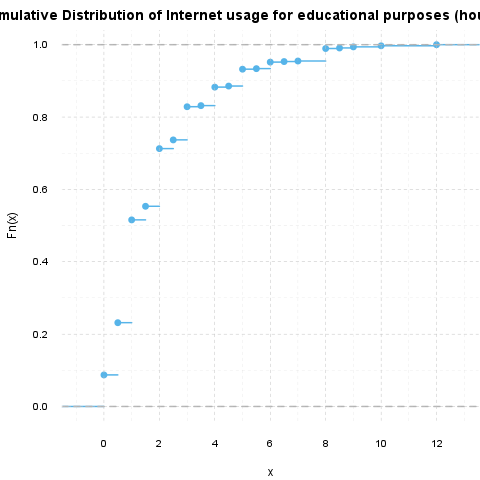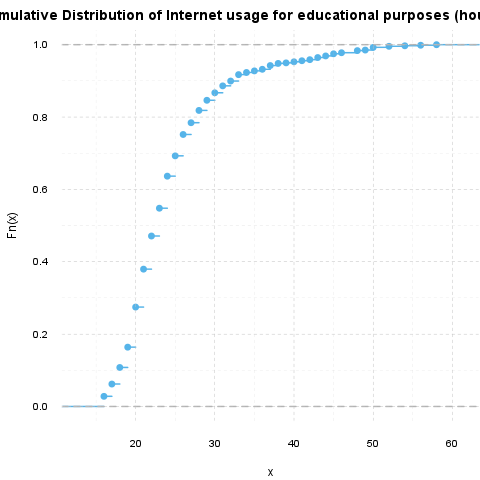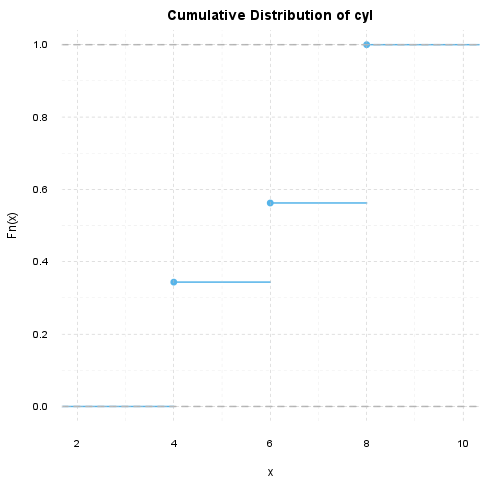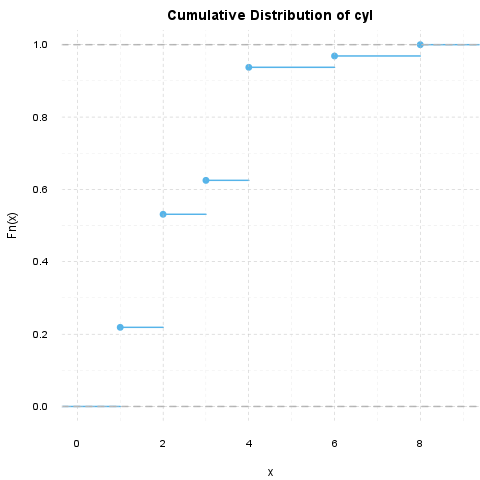## Description

This template will run a Kolmogorov-Smirnov-test

#### Introduction

Kolmogorov-Smirnov test is one of the most widely used nonparametric tests. With the help of that in this case we use to check if two continuous variables had the same distribution. We do not test that here, but there is a possibility to use that in the way to check if a sample/variable followed an expected distribution.

#### Distributions

Before we use the K-S test to look at the possible statistical differences, it could be useful to see visually the distributions we want to observe. Below lie the Cumulative Distribution Functions of the variables we compared:#### Test results

Now we will test if the Internet usage for educational purposes (hours per day) and the Age had statistically the same distribution.

Two-sample Kolmogorov-Smirnov test on Internet usage for educational purposes (hours per day) and Age
Test statistic P value Alternative hypothesis
1 0 * * * two-sided

The requirements of the Kolmogorov-Smirnov Test test was not met, the approximation may be incorrect.

So the variables do not follow the same distribution, according to the Kolmogorov-Smirnov test statistic.

## Description

This template will run a Kolmogorov-Smirnov-test

#### Introduction

Kolmogorov-Smirnov test is one of the most widely used nonparametric tests. With the help of that in this case we use to check if two continuous variables had the same distribution. We do not test that here, but there is a possibility to use that in the way to check if a sample/variable followed an expected distribution.

#### Distributions

Before we use the K-S test to look at the possible statistical differences, it could be useful to see visually the distributions we want to observe. Below lie the Cumulative Distribution Functions of the variables we compared:#### Test results

Now we will test if the cyl and the carb had statistically the same distribution.

Two-sample Kolmogorov-Smirnov test on cyl and carb
Test statistic P value Alternative hypothesis
0.625 7.453e-06 * * * two-sided

The requirements of the Kolmogorov-Smirnov Test test was not met, the approximation may be incorrect.

So the variables do not follow the same distribution, according to the Kolmogorov-Smirnov test statistic.

This report was generated with R (3.0.1) and rapport (0.51) in 0.729 sec on x86_64-unknown-linux-gnu platform.